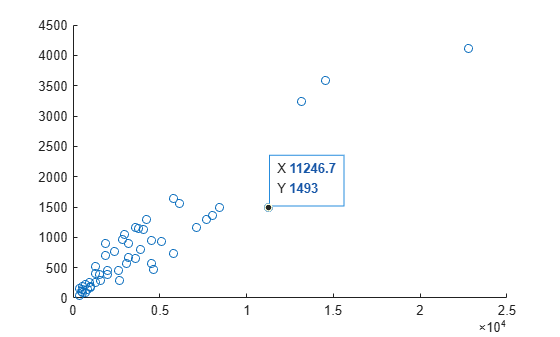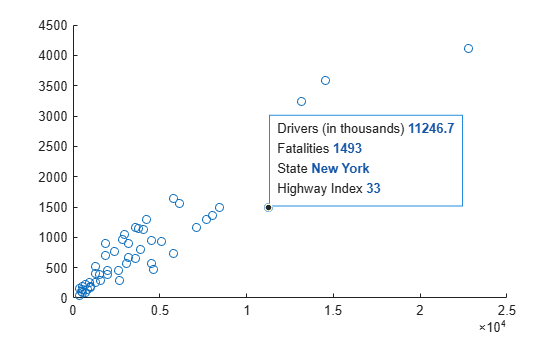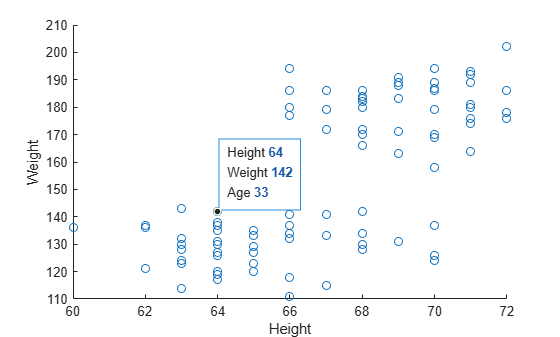## Create Custom Data Tips

Data tips appear when you hover over a data point. By default, data tips show the coordinates of the selected point. However, for some types of charts, you can customize the information that appears in the data tip, such as changing the labels or adding new rows.

Charts that support these customizations have a `DataTipTemplate` property, for example, `Line` objects created with the `plot` function.

### Change Labels and Add Row

Modify the contents of data tips on a scatter plot. First, load sample accident data and create the scatter plot. Then, create a data tip interactively or by using the `datatip` function. By default, data tips show the coordinates of the data point.

```load('accidents.mat','hwydata','statelabel') s = scatter(hwydata(:,5),hwydata(:,4)); dt = datatip(s,11250,1493);```Change the data tip labels from `X` and `Y` to `Drivers (in thousands)` and `Fatalities` by accessing the `DataTipTemplate` property of the plotted object and setting the `Label` properties.

```s.DataTipTemplate.DataTipRows(1).Label = 'Drivers (in thousands)'; s.DataTipTemplate.DataTipRows(2).Label = 'Fatalities'; ```

Add a new row to the data tip. For the label, use `State`. For the value, use the state names contained in the `statelabel` variable in your workspace.

```row = dataTipTextRow('State',statelabel); s.DataTipTemplate.DataTipRows(end+1) = row;```### Show Table Values in Data Tips

Modify the contents of data tips for a scatter plot to include values from a table. First, create a table from a sample spreadsheet of patient data. Plot the data. Then, create a data tip interactively or by using the `datatip` function.

```tbl = readtable('patients.xls'); s = scatter(tbl.Height,tbl.Weight); dt = datatip(s,64,142);```

Change the data tip labels from `X` and `Y` to `Height` and `Weight`. Then, add a new row to the data tip that uses the label `Age` and shows the values from the Age column of the table.

```s.DataTipTemplate.DataTipRows(1).Label = 'Height'; s.DataTipTemplate.DataTipRows(2).Label = 'Weight'; row = dataTipTextRow('Age',tbl.Age); s.DataTipTemplate.DataTipRows(end+1) = row;```If you are using R2018b or earlier, customize the data tips by setting the `UpdateFcn` property of the `datacursormode` object instead of using the `DataTipTemplate` object.